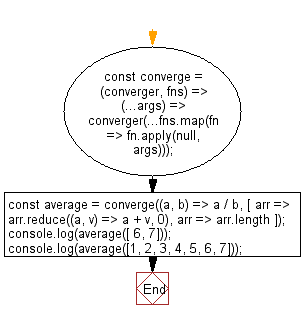# JavaScript: Accepts a converging function and a list of branching functions

## JavaScript fundamental (ES6 Syntax): Exercise-68 with Solution

Write a JavaScript program that accepts a converging function and a list of branching functions and returns a function that applies each branching function to the arguments and the results of the branching functions are passed as arguments to the converging function.

• Use Array.prototype.map() and Function.prototype.apply() to apply each function to the given arguments.
• Use the spread operator (...) to call converger with the results of all other functions.

Sample Solution:

JavaScript Code:

``````//#Source https://bit.ly/2neWfJ2
const converge = (converger, fns) => (...args) => converger(...fns.map(fn => fn.apply(null, args)));
const average = converge((a, b) => a / b, [
arr => arr.reduce((a, v) => a + v, 0),
arr => arr.length
]);
console.log(average([ 6, 7]));
console.log(average([1, 2, 3, 4, 5, 6, 7]));
```
```

Sample Output:

```6.5
4
```

Pictorial Presentation:Flowchart:Live Demo:

See the Pen javascript-basic-exercise-68-1 by w3resource (@w3resource) on CodePen.

Improve this sample solution and post your code through Disqus

What is the difficulty level of this exercise?

Test your Programming skills with w3resource's quiz.

﻿

## JavaScript: Tips of the Day

Returns the unique symmetric difference between two arrays, not containing duplicate values from either array

Example:

```const uniqueSymmetricDifference = (a, b) => [
...new Set([...a.filter(v => !b.includes(v)), ...b.filter(v => !a.includes(v))])
];
console.log(uniqueSymmetricDifference([1, 2, 3], [1, 2, 4])); // [3, 4]
console.log(uniqueSymmetricDifference([1, 2, 2], [1, 3, 1])); // [2, 3]
```

Output:

```[3, 4]
[2, 3]
```